# Histograms (Basics)

GCSELevel 4-5Cambridge iGCSE

## Histograms (Basics)

Histograms are a way to represent continuous data from a grouped frequency table.

The class widths can vary in size, however on this page only histograms with equal class widths will be discussed. Students sitting the higher exam should refer to this page on histograms.

Even though histograms with equal class width can look very similar to bar charts, there is actually $1$ important difference, which is that the bars do not have gaps in between them.

Make sure you are happy with the following topics before continuing.

## Constructing Histograms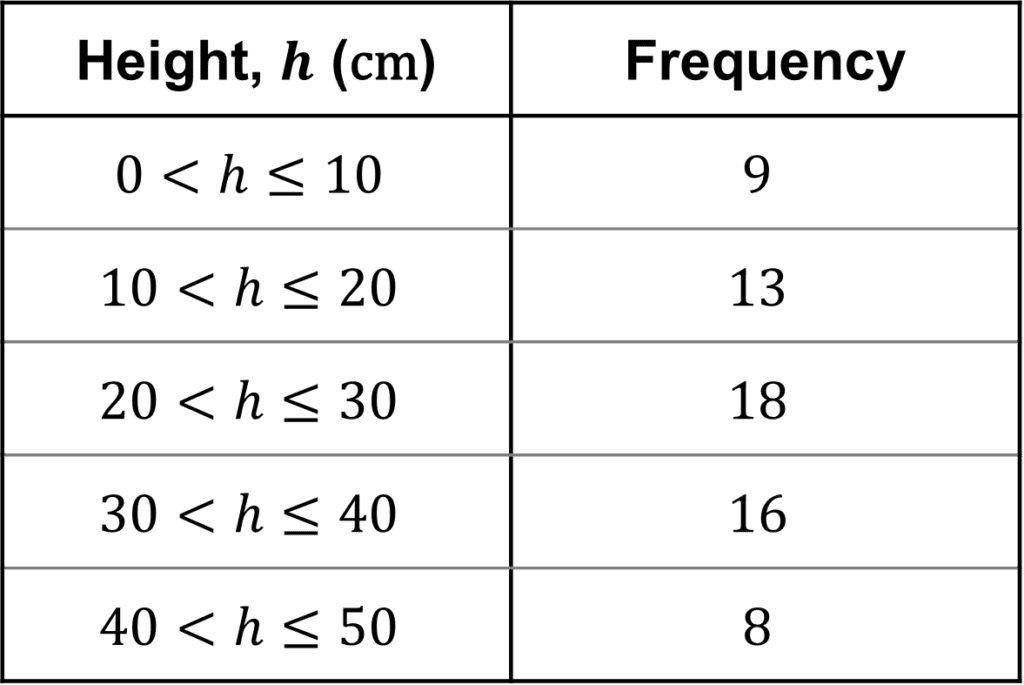As mentioned above, histograms are used to display continuous data, which will be given to you in the form of a grouped frequency table. The grouped frequency table on the right shows the height of some different plants.

Using the data in the table, then choose a suitable scale for your $x$ and $y$ axes.

The $x$-axis will display the different classes, which in this case is the heights of some plants. The $y$-axis will display the corresponding frequencies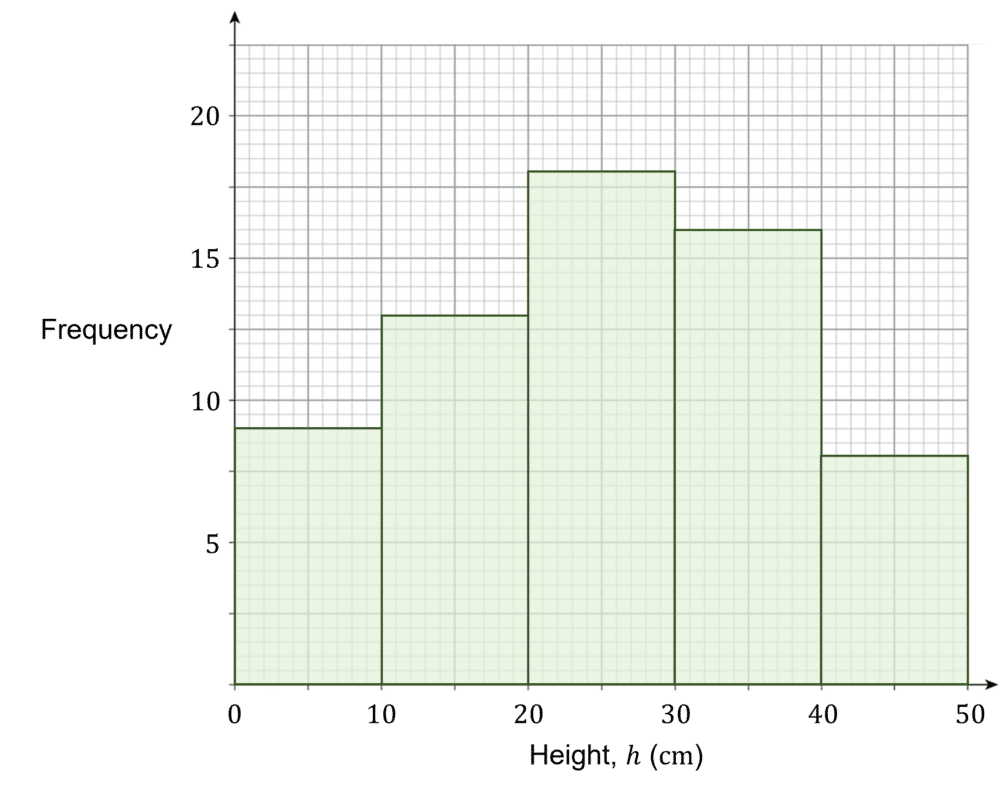Level 4-5GCSECambridge iGCSE

## Example 1: Reading from a Histogram

The histogram below, shows the speeds of different cars driving through a $30 \text{ mph}$ zone. Use the histogram to complete the grouped frequency table.

[2 marks]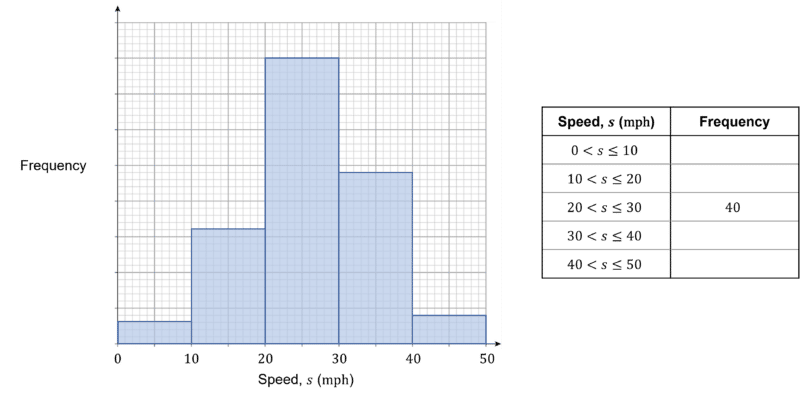The middle bar is $8$ units tall, therefore $1$ unit $=40\div8=5$ students.

This can then be used to complete the values for the $y$-axis, as shown below.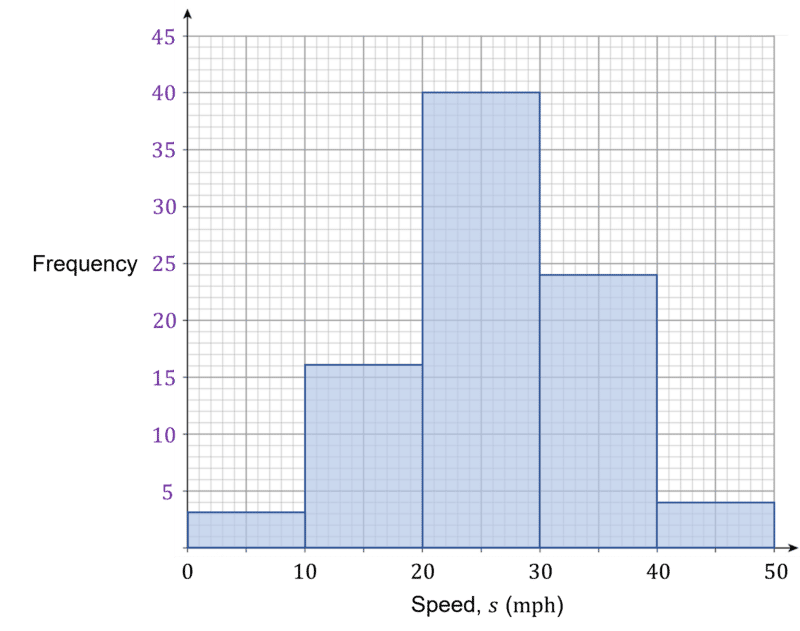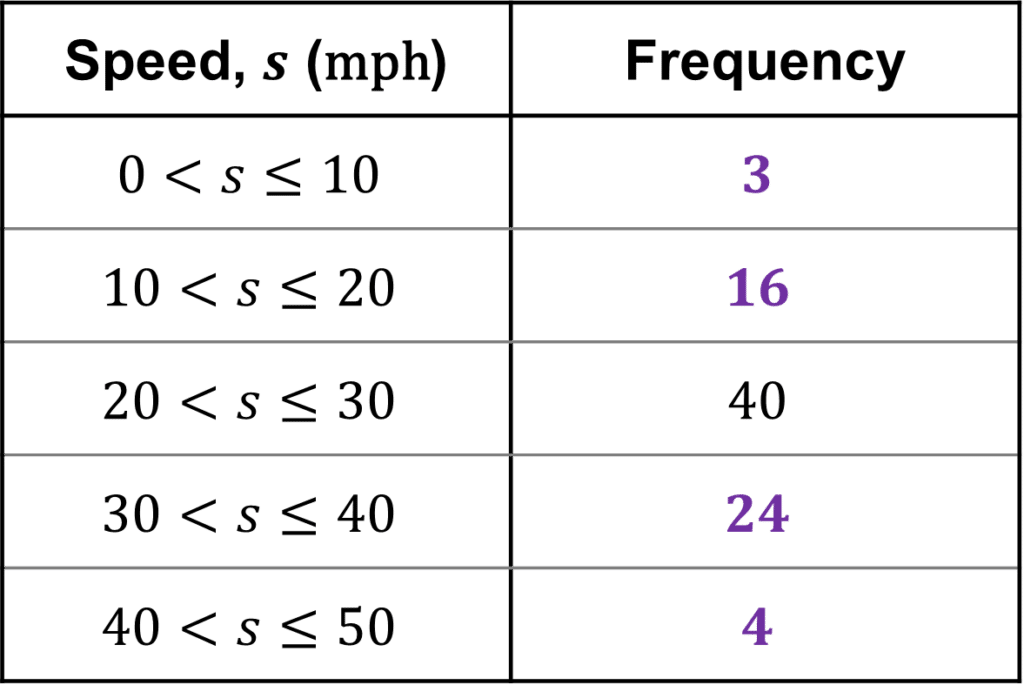Now simply read off the frequencies to complete the table.

Level 4-5GCSECambridge iGCSE

## Example 2: Estimating from a Histogram

The histogram shown below shows the weight of some different students in year $10$.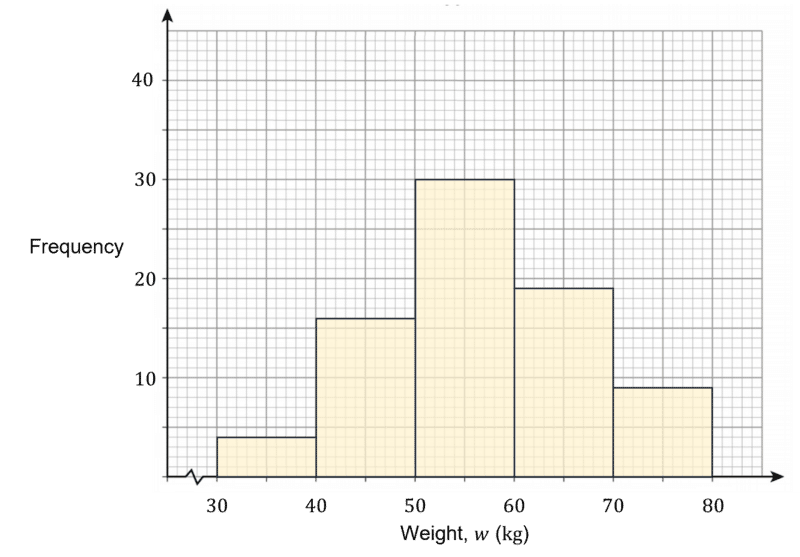Use the histogram to estimate the amount of students who weigh more than $58 \text{ kg}$.

[2 marks]

To estimate the amount of students heavier than $58 \text{ kg}$, we can draw a vertical line from $58 \text{ kg}$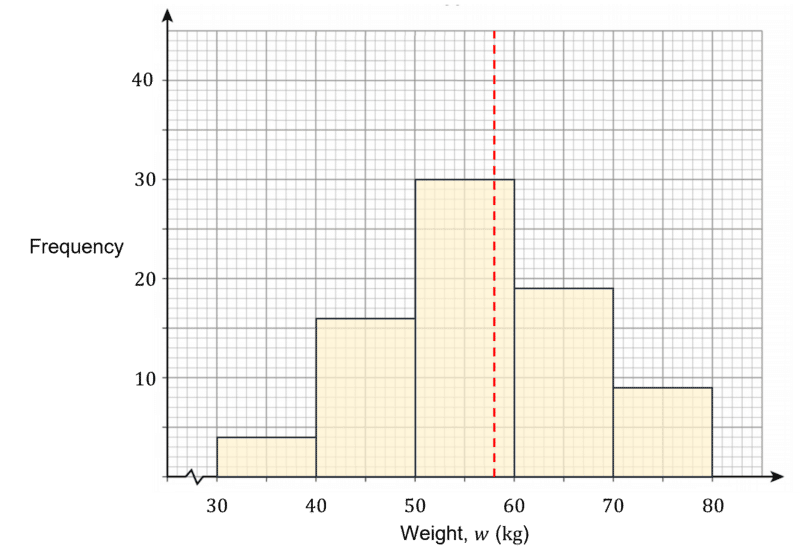Using this line, we can see that $\dfrac{2}{10}$ of the $50 bar and all of the $60 and $70 bars are above $58 \text{ kg}$.

Reading from the histogram, the estimate for the amount of students heavier than $58 \text{ kg}$ is:

$\dfrac{2}{10}\times 30 + 19 + 9=34$

Note: This is only an estimate, as we are not given the specific weights of individual students within each class width.

Level 4-5GCSECambridge iGCSE

## Histograms (Basics) Example Questions

Choosing a suitable scale for both the $x$ and $y$ axes, your histogram should look similar to this: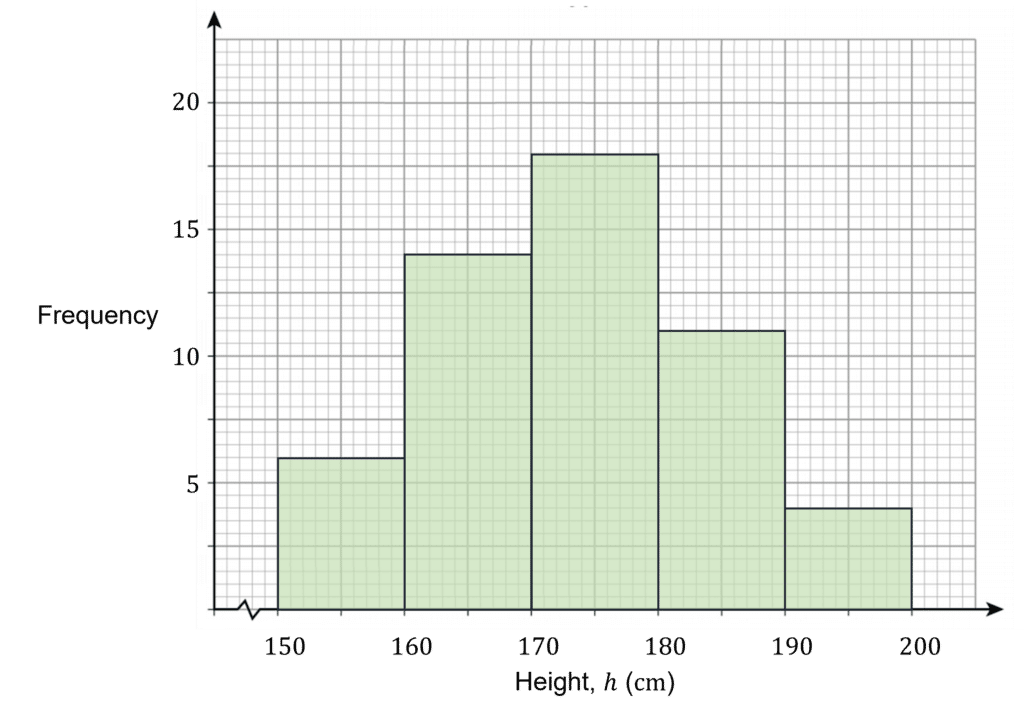Note: The squiggle or sideways ‘Z’ on the $x$-axis, shows that part of the scale has been cut off. This is because starting at $0$ wouldn’t be practical, given the minimum possible value is $150 \text{ cm}$.

The first bar is $2.4$ units tall, so $1$ unit $=12\div2.4=5$ people.

The $y$ axis scale can then be added as shown below.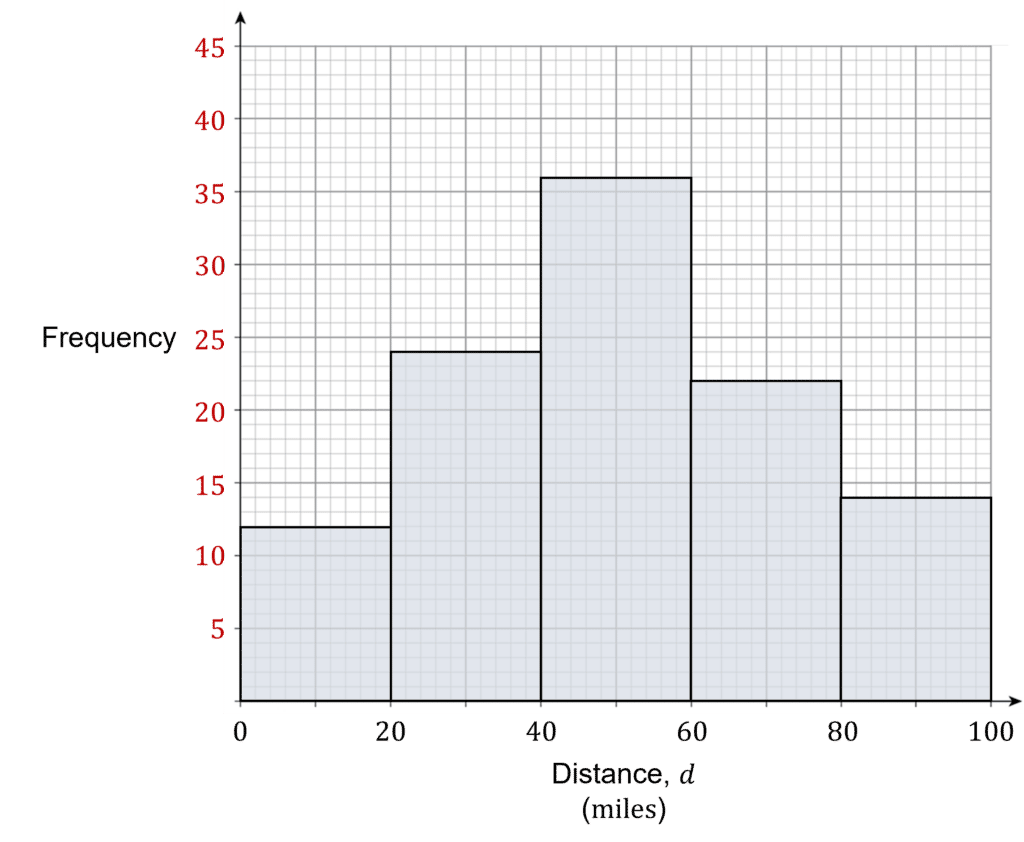Reading off the histogram, the grouped frequency table can then be completed.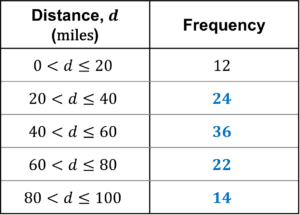Using the histogram, we can see that $\dfrac{1}{5}$ of the $5 is above $9\degree \text{C}$, as well as the bars $10, $15 and $20.

Therefore we can estimate that:

$\dfrac{1}{5}\times25+37+14+4=60$ days were above the yearly average in August, September and October.

## You May Also Like...### MME Learning Portal

Online exams, practice questions and revision videos for every GCSE level 9-1 topic! No fees, no trial period, just totally free access to the UK’s best GCSE maths revision platform.

£0.00